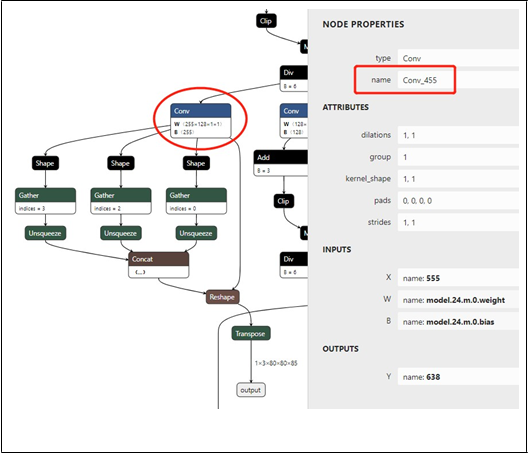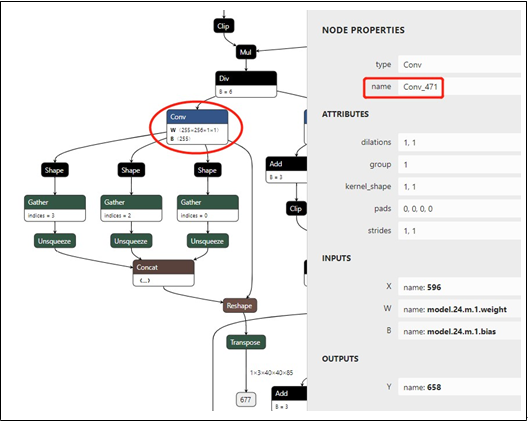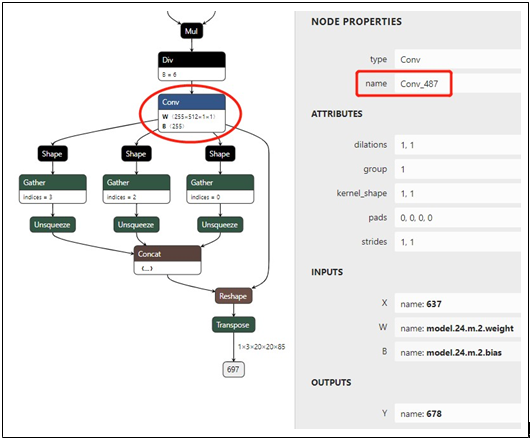### 使用 OpenVINO™ 运行 YOLOv5 推理openlab_4276841a 更新于 6月前

### 2.0 将权重转换为 ONNX 文件

OpenVINO™ - 在本文档中，我们使用 OpenVINO™ Linux* 版本 2021.1 版进行验证

Python* – 在本文档中，我们使用 Python 3.6.9 进行验证
ONNX – 在本文档中，我们使用 ONNX 1.6.0 进行验证
PyTorch – 在本文档中，我们使用 PyTorch 1.6.0 进行验证
Netron* – 在本文档中，我们使用 Netron 4.4.3 进行验证。

#### 2.1. 从 GitHub 复制 YOLOv5 存储库

``\$ git clone https://github.com/ultralytics/yolov5``

#### 2.2. 设置 YOLOv5 的环境

``\$ pip install -r requirements.txt\$ pip install onnx``

#### 2.3. 下载 PyTorch 权重

``\$ wget https://github.com/ultralytics/yolov5/releases/download/v3.0/yolov5s.pt``

#### 2.4. 将 PyTorch 权重转换为 ONNX 权重

YOLOv5 存储库提供了 models/export.py 脚本，您可以使用该脚本将带 *.pt 扩展名的 PyTorch 权重导出为带 *.onnx 扩展名的 ONNX 权重

``torch.onnx.export(model, img, f, verbose=False, opset_version=10, input_names=['image******r>                                  output_names=['classe******oxes'] if y is None else ['out put'])``

``\$ python models/export.py	--weights yolov5-v3/yolov5s.pt	--img 640 -- batch 1``

### 3.0 将 ONNX 文件转换为 IR 文件

``\$ source /opt/intel/openvino_2021/bin/setupvars.sh``YOLOv5 中有 3 个输出节点。我们使用 Netron 查看 YOLOv5 ONNX 权重。然后通过在 Netron 中搜索关键词“Transpose”找到输出节点。随后，我们可以查找卷积节点，该节点在图 1. 中被标记为椭圆形。双击节点后，我们可以在属性面板中读取其名称“Conv_455”，名称在 图 1 中被标记为矩形。图 1 显示大小为 1x3x80x80x85 的输出节点和分辨率为 1x3x640x640 的输入图像，用于检测小型对象。 我们使用卷积节点的名称“Conv_455”来指定模型优化器参数。``\$ python/opt/intel/openvino_2021.1.110/deployment_tool***odel_optimizer/mo.py--input_model yolov5-v3/yolov5s.onnx --model_name yolov5-v3/yolov5s -***r>255 --reverse_input_channels --output Conv_487,Conv_471,Conv_455``### 4.0 推理 Python 演示

1) 使用 letterbox 预处理输入图像

2) 使用 Sigmoid 函数的 YOLO region 层

3) 边界框后处理

#### 4.1. 使用 letterbox 预处理输入图像

YOLOv5 使用 letterbox 处理网络的输入图像。在 YOLOv5 推理 Python 演示中，我们提供了一个用于处理图像的函数：

``def letterbox(img, size=(640, 640), color=(114, 114, 114), auto=True, scaleFill=False,scaleup=True):# Resize image to a 32-pixel-multiple rectangle https://github.com/ultralytics/yolov3/issues/232shape = img.shape[:2] # current shape [height, width]w, h = size# Scale ratio (new / old)r = min(h / shape, w / shape)if not scaleup: # only scale down, do not scale up (for better test mAP)r = min(r, 1.0)# Compute paddingratio = r, r # width, height ratio***r>new_unpad = int(round(shape * r)), int(round(shape * r))dw, dh = w - new_unpad, h - new_unpad # wh paddingif auto: # minimum rectangledw, dh = np.mod(dw, 64), np.mod(dh, 64)  # wh paddingelif scaleFill: # stretch dw, dh = 0.0, 0.0 new_unpad = (w, h)dw /= 2 # divide padding into 2 side***r>dh /= 2if shape[::-1] != new_unpad: # resizeimg = cv2.resize(img, new_unpad, interpolation=cv2.INTER_LINEAR) top, bottom = int(round(dh - 0.1)), int(round(dh + 0.1))left, right = int(round(dw - 0.1)), int(round(dw + 0.1))img = cv2.copyMakeBorder(img, top, bottom, left, right, cv2.BORDER_CONSTANT, value=color) # add bordertop2, bottom2, left2, right2 = 0, 0, 0, 0if img.shape != h:top2 = (h - img.shape)//2 bottom2 = top2img = cv2.copyMakeBorder(img, top2, bottom2, left2, right2, cv2.BORDER_CONS TANT, value=color)  # add borderelif img.shape != w:left2 = (w - img.shape)//2right2 = left2img = cv2.copyMakeBorder(img, top2, bottom2, left2, right2, cv2.BORDER_CONS TANT, value=color)  # add borderreturn img``

#### 4.2. 使用 Sigmoid 函数的 YOLO Region 层

``def parse_yolo_region(blob, resized_image_shape, original_im_shape, params, thres hold):# ------------------------------------------ Validating output parameter**********************************************r>out_blob_n, out_blob_c, out_blob_h, out_blob_w = blob.shapepredictions = 1.0/(1.0+np.exp(-blob))assert out_blob_w == out_blob_h, "Invalid size of output blob.It should be in NCH W layout and height should " \"be equal to width.Current height = {}, current width = {}" \"".format(out_blob_h, out_blob_w)# ------------------------------------------ Extracting layer parameter***********************************************r>orig_im_h, orig_im_w = original_im_shaperesized_image_h, resized_image_w = resized_image_shapeobjects = list()side_square = params.side * params.side# ------------------------------------------- Parsing YOLO Region output -------------------------------------------bbox_size = int(out_blob_c/params.num) #4+1+num_classe***r>for row, col, n in np.ndindex(params.side, params.side, params.num):bbox = predictions[0, n*bbox_size:(n+1)*bbox_size, row, col]x, y, width, height, object_probability = bbox[:5]class_probabilitie*****box[5:]if object_probability < threshold: continuex = (2*x - 0.5 + col)*(resized_image_w/out_blob_w) y = (2*y - 0.5 + row)*(resized_image_h/out_blob_h)if int(resized_image_w/out_blob_w) == 8 & int(resized_image_h/out_blob_h) == 8: #80x80,idx = 0elif int(resized_image_w/out_blob_w) == 16 & int(resized_image_h/out_blob_h)== 16: #40x40idx = 1elif int(resized_image_w/out_blob_w) == 32 & int(resized_image_h/out_blob_h)== 32: # 20x20idx = 2width = (2*width)**2* params.anchors[idx * 6 + 2 * n]height = (2*height)**2 * params.anchors[idx * 6 + 2 * n + 1] class_id = np.argmax(class_probabilitie****r>confidence = object_probabilityobjects.append(scale_bbox(x=x, y=y, height=height, width=width, class_id=clas***r>_id, confidence=confidence,im_h=orig_im_h, im_w=orig_im_w, resized_im_h=resized_image_h, resized_im_w=resized_image_w)) return objects``

#### 4.3. 边界框后处理

``def scale_bbox(x, y, height, width, class_id, confidence, im_h, im_w, resized_im_h=640, resized_im_w=640):gain = min(resized_im_w / im_w, resized_im_h / im_h) # gain = old / newpad = (resized_im_w - im_w * gain) / 2, (resized_im_h - im_h * gain) / 2 # wh paddi ngx = int((x - pad)/gain)y = int((y - pad)/gain)w = int(width/gain)h = xmin = max(0, int(x - w / 2))ymin = max(0, int(y - h / 2)) xmax = min(im_w, int(xmin + w)) ymax = min(im_h, int(ymin + h))# Method item() used here to convert NumPy types to native types for compatibility with functions, which don't# support Numpy types (e.g., cv2.rectangle doesn't support int64 in color parameter)return dict(xmin=xmin, xmax=xmax, ymin=ymin, ymax=ymax, class_id=class_id.ite m(), confidence=confidence.item())``

### 5.0 NCS2 的准确度下降问题

``\$ git clone https://github.com/openvinotoolkit/openvino``

``\$ cd openvino\$ git submodule update --init --recursive\$ git checkout -b yolov5 2021.1``

``if (firstStage && shift < 4 && isGrowingOutput && weights->desc().dim(Dim::C)	> 1) {normalVal = 5;shift = correctShift(shift, firstStage, stage->origLayer()->type); shift -= normalVal;}//shift = correctShift(shift, firstStage, stage->origLayer()->type);//shift -= normalVal;``

``\$ mkdir build & cd build\$ cmake -DCMAKE_BUILD_TYPE=Release -DENABLE_PYTHON=ON -DPYTHON_EXECUTABLE=`which python3.6`	- DPYTHON_LIBRARY=/usr/lib/x86_64-linux-gnu/libpython3.6m.so	- DPYTHON_INCLUDE_DIR=/usr/include/python3.6 ..\$ make --jobs=\$(nproc --all)``

0个评论